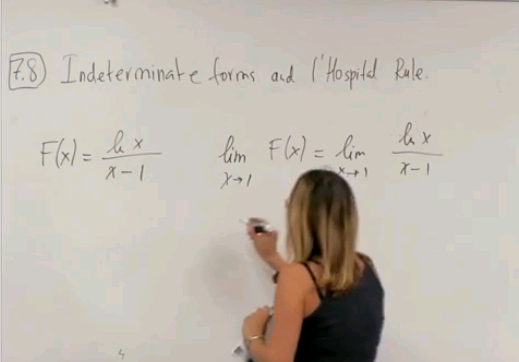# Home

## Math 2B. Lec. 15. Calculus: Indeterminate Forms and L'Hospital Rule (English)

UCI Math 2B: Single-Variable Calculus (Fall 2013)
Lec 15. Single-Variable Calculus -- Indeterminate Forms and L'Hospital Rule --
View the complete course: http://ocw.uci.edu/courses/math_2b_calculus.html
Instructor: Natalia L. Komarova Ph.D.

More courses at http://ocw.uci.edu

Description: UCI Math 2B is the second quarter of Single-Variable Calculus and covers the following topics: Definite integrals; the fundamental theorem of calculus. Applications of integration including finding areas and volumes. Techniques of integration. Infinite sequences and series. Parametric and polar equations.

Recorded on November 1, 2013

Required attribution: Komarova, Natalia L. Math 2B (UCI OpenCourseWare: University of California, Irvine), http://ocw.uci.edu/courses/math_2b_calculus.html. [Access date]. License: Creative Commons Attribution-ShareAlike 3.0 United States License.Author:
Natalia Komarova
Title:
Professor
Department:
Mathematics